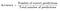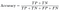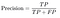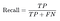# What is Accuracy, Precision, and Recall? And Why are they Important?

Understanding how to assess the efficacy of your model is imperative. If you don’t understand how to interpret the results of a classification model then it will preclude you from being able to improve upon that model and properly implement it for the task that it is setting out to achieve.

When it comes to Linear Regression, typical metrics that are used to assess the model are : MSE , RMSE, MAE etc. These measures of error should be intuitive, despite using different mechanisms. They all capture or represent the extent to which the predicted values of the model deviate form the actual values. Well, then what do we do when our model is a classification one and not a regression one? We use metrics such as Accuracy, Precision, Recall, Sensitivity, and F1!

At a first glance these metrics may appear to be confusing and difficult to conceptualize, but they are actually straightforward. What is often confusing is the nomenclature or the names assigned to these metrics. My suggestion is to not associate the colloquial or lexical ( dictionary ) definition of these words to their meaning in the context of machine learning or statistics. In machine learning and statistics, these terms are technical and have a very specific meaning that don’t necessarily coincide with our everyday use of the words “precision”, “accuracy”, and “sensitivity”. Another source of confusion is the similarity among some of the formulas and understanding the distinction and difference between what they pragmatically measure.

Before Defining these classification metrics, it is critical to understand what TP, FP, FN, and TN mean. T (True) means that the model has correctly classified an observation. In other words, the predicted class and actual class coincide with one another. F (False) means that the model has incorrectly classified an observation by assigning it to the wrong class. TP ( True Positive) means the model was correctly classified in the positive class. TN ( True Negative) means the model correctly classified an observation in the negative class. FP (False positive) means the model classified an observation to be positive when in reality it was actually negative. FN ( False Negative) means the model incorrectly classified an observation as negative when it should have been classified as positive.

# AccuracyAccuracy Formula

The accuracy of a machine learning classification algorithm is one way to assess how often model classifies a data point correctly. The numerator is total number of predictions that were correct. The denominator is the total number of predictions. The numerator will only include TP and TN and the denominator will be include TP, TN, FP, and FN. Accuracy is a ratio of the correctly classified data to the total amount of classifications made by the model.

For Binary Classification the formula for accuracy precisely is:Accuracy for Binary Classification

Let’s try using accuracy for the model below that classified one hundred tumors as either malignant (positive class) or benign ( negative class).

From the 100 tumors that were classified, 91 were correctly classified (0.91 or 91 %). Does this mean that the tumor classifier is doing an excellent job of identifying malignancies?

Well, let’s do a closer analysis of positives and negatives to gain more insight into our model’s performance.

Of the 100 tumor examples, 91 are benign (90 TNs and 1 FP) and 9 are malignant (1 TP and 8 FNs).

Of the 91 benign tumors, the model correctly identifies 90 as benign. That’s good. However, of the 9 malignant tumors, the model only correctly identifies 1 as malignant — a terrible outcome, as 8 out of 9 malignancies go undiagnosed!

While 91% accuracy may seem good at first glance, another tumor-classifier model that always predicts benign would achieve the exact same accuracy (91/100 correct predictions) on our examples. In other words, our model is no better than one that has zero predictive ability to distinguish malignant tumors from benign tumors.

Accuracy alone doesn’t tell the full story when you’re working with a class-imbalanced data set, like this one, where there is a significant disparity between the number of positive and negative labels.

It’s also important to understand what we would like to optimize. In this context it is better to have an improved false negative score than an improved false positive score. Telling someone they don’t have cancer when they actually do is more detrimental to telling someone that they have cancer when they actually don’t. This is so because if you tell someone they don’t have cancer when they actually do then they can go untreated as the cancer progresses, whereas in the other situation more medical tests will be administered to confirm the diagnosis.

In order to help with this situation let’s examine Precision and Recall!

# Precision and Recall

Precision and Recall are concepts that are related but have an important distinction. It is crucial that this difference is understood, so you can improve your model to optimize for a specific metric.

Precision attempts to answers the question: What proportion of positive identifications was actually correct?

Precision is defined as follows:Precision Formula

From the above problem with tumors, let’s attempt to calculate the precision

The model has a precision score of 50 %, which means that when it classifies a tumor to be malignant it is correct 50 % of the time.

Recall attempts to answer the question: What proportion of actual positives was identified correctly?Recall Formula

Let’s calculate the recall value for the tumor classifier model

The recall is 11 %, which means it correctly classifies only 11 % of the malignant tumors.

This demonstrates that Accuracy, although a great metric, is very limited in its scope and can be deceiving. It is always crucial to calculate the precision and recall and not to stop after accuracy. It is also important to understand when to optimize for precision and went to optimize for recall.

Well Why cant we always improve both?

Precision and Recall are often in collision. Meaning, improving one score can come at the cost of decreasing the other. So the decision to improve recall or precision is situational and depends heavily on the type of problem that is being solved. In the context of diagnostics and medicine, it is important to improve recall because, as already mentioned, it is better to classify someone as being positive for cancer when they don’t have cancer as opposed to the converse. There are situations in which we would like to increase precision in place of recall. For example, if we were building a classification model that classified emails as spam or not spam. It is better to have an email classified as not spam when it is actually spam then have an email classified as spam when it actually isn’t.

# Conclusion

Accuracy, Precision, and Recall are all critical metrics that are utilized to measure the efficacy of a classification model. Accuracy is a good starting point in order to know the number of correctly predicted values in relation to the total prediction values, but it is important to understand that further metrics must be implemented such as Recall and Precision. Understanding the heart of the task will dictate whether you should focus on improving Recall or Precision.

## More from Shiffraw dagnechaw

Data Science Enthusiast